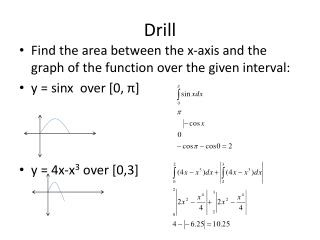DownloadDownload PresentationDrill

# Drill

Download Presentation## Drill

- - - - - - - - - - - - - - - - - - - - - - - - - - - E N D - - - - - - - - - - - - - - - - - - - - - - - - - - -
##### Presentation Transcript

1. Drill • Find the area between the x-axis and the graph of the function over the given interval: • y = sinx over [0, π] • y = 4x-x3 over [0,3]

2. 7.2: Applications of Definite Integrals Day #1 Homework: page 395-396 (1-10) Day #2 Homework: Page 396-397, 11-14, 27-37 (odd)

3. What you’ll learn about… • Area between curves • Ares enclosed by intersecting curves • Boundaries with changing functions • Integrating with respect to y • Saving time with geometric formulas.

4. Area Between Curves • If f and g are continuous with f(x) > g(x) through [a,b], then the area between the curves y = f(x) and y = g(x) from a to b is the integral of [f-g] from a to b,

5. Applying the Definition • Find the area of the region between the and y = sec2x and y = sinx from x = 0 to x = π/4. • When graphed, you can see that y = sec2 x is above y = sinx on [0, π/4].

6. Area of an Enclosed Region • When a region is enclosed by intersecting curves, the intersection points give the limits of integration. • Find the area of the region enclosed by the parabola y = 2 – x2 and the line y = -x. • Solution: graph the curves to determine the x-values of the intersections and if the parabola or the line is on top.

7. Using a Calculator • Find the area of the region enclosed by the graphs of y = 2cosx and y = x2-1. • Solution: Graph to determine the intersections. Don’t forget to use Zoom 7: Trig (radian mode, please) • X = ± 1.265423706 • fnInt(2cosx –(x2-1), x, -1.265423706, 1.265423706) • 4.994 units2

8. Boundaries with Changing Functions • If a boundary of a region is defined by more than one function, we can partition the region into subregions that correspond to the function changes. • Find the area of the region R in the first quadrant bounded by y = (x)1/2 and below by the x-axis AND the line y = x -2 Region A: Region B: B A A +B = 3.33

9. Drill: Find the x and y coordinates of all points where the graphs of the given functions intersect • y = x2 – 4x and x + 6 • y = ex and y = x + 1 • y = x2 – πx and y = sin x • (-1, 5) and (6, 12) • (0,1) • (0,0)and (3.14, 0)

10. Integrating with Respect to y • Sometimes the boundaries of a region are more easily described by functions of y than by functions of x. We can use approximating rectangles that are horizontal rather than vertical and the resulting basic formula has y in place of x.

11. Integrating with Respect to y • Find the area of the region R in the first quadrant bounded by y = (x)1/2 and below by the x-axis AND the line y = x -2 by integrating with respect to x. • y = x – 2 becomes y + 2 = x and y = (x)1/2becomes y2 = x, y > 0

12. 10/3 or 3.3333333

13. Making the Choice • Find the area of the region enclosed by the graph y = x3 and x = y2 – 2 (To graph…. • It makes more sense to integrate with respect to y because you will not have to split the region. • The lower limit is when the y – value = -1 • The upper limit needs to be found in the calc. y = 1.79

14. Solution • Solve both equations for x. • y1/3= x and x = y2 -2 • Set up your definite integral: right – left • Evaluate using the calculator. • fnInt(x1/3 – x2 + 2, x, -1, 1.79) • 4.21 units2

15. Using Geometry • Another way to complete find the area of the region R in the first quadrant bounded by y = (x)1/2 and below by the x-axis AND the line y = x -2 by integrating with respect to x. • You can find the area under y = x to the x-axis from 0 to 4 and then subtract the area of the triangle formed by the line y = x -2 and the x-axis. 10/3 units2